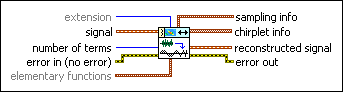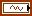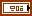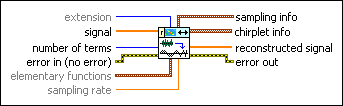LabVIEW 2014 Advanced Signal Processing Toolkit Help

Edition Date: June 2014

Part Number: 372656C-01

»View Product InfoDownload Help (Windows Only)

Owning Palette: Time Frequency Transform VIs

Decomposes signal as a linear combination of Gaussian chirplet elementary functions. Wire data to the signal input to determine the polymorphic instance to use or manually select the instance.

Examples

Use the pull-down menu to select an instance of this VI.extension specifies the method to use to pad data at the borders of the input signal to lessen discontinuity. The extension length is half the window length.

 0 Zero padding (default)—Uses zeros to pad the input data. Watch for abrupt transitions between the padded zeros and the input data, which causes large artifacts near the transition. 1 Symmetric—Uses replications of the input data to pad the data, except that this VI left-flips the block at the input and right-flips the block at the end. 2 Periodic—Adds a replication of the input data block before and another replication after the input data block to pad the data.signal specifies the input signal.number of terms specifies the maximum number of elementary functions to use in the adaptive representation. Using more elementary functions improves the accuracy of the representation but increases computation time.error in describes error conditions that occur before this node runs. This input provides standard error in functionality.elementary functions specifies the settings of the elementary functions.type specifies the type of elementary function to use to represent the signal.

 0 Gaussian chirplet (default)—Specifies that the elementary function is the Gaussian chirplet. 1 Gaussian pulse—Specifies that the elementary function is the Gaussian pulse.max window length specifies the maximum window length of the Gaussian envelopes of the elementary functions. The default is –1, which specifies that this VI sets the maximum window length as the power of 2 that is closest to but less than the signal length. The value of max window length must be a power of 2. If the value of max window length is not a power of 2, this VI sets the value to a power of 2 automatically. Use the default setting if the size of the input signal is not greater than 1,000,000.reserved is reserved for future use.sampling info returns the sampling information of the signal.sampling rate returns the sampling rate of the signal.length returns the length, in samples, of the signal.chirplet info returns the parameters of each detected Gaussian chirplet elementary function.amplitude returns the complex amplitude of the chirplet or Gaussian pulse.standard deviation returns the standard deviation of the Gaussian envelope in seconds.center returns the central time of the Gaussian envelope in seconds.frequency returns the central frequency of the chirplet in hertz.chirp rate returns the sweep rate of the carrier sine wave in hertz per second.reconstructed signal returns the signal reconstructed from the detected elementary functions.error out contains error information. This output provides standard error out functionality.extension specifies the method to use to pad data at the borders of the input signal to lessen discontinuity. The extension length is half the window length.

 0 Zero padding (default)—Uses zeros to pad the input data. Watch for abrupt transitions between the padded zeros and the input data, which causes large artifacts near the transition. 1 Symmetric—Uses replications of the input data to pad the data, except that this VI left-flips the block at the input and right-flips the block at the end. 2 Periodic—Adds a replication of the input data block before and another replication after the input data block to pad the data.signal specifies the input signal.number of terms specifies the maximum number of elementary functions to use in the adaptive representation. Using more elementary functions improves the accuracy of the representation but increases computation time.error in describes error conditions that occur before this node runs. This input provides standard error in functionality.elementary functions specifies the settings of the elementary functions.type specifies the type of elementary function to use to represent the signal.

 0 Gaussian chirplet (default)—Specifies that the elementary function is the Gaussian chirplet. 1 Gaussian pulse—Specifies that the elementary function is the Gaussian pulse.max window length specifies the maximum window length of the Gaussian envelopes of the elementary functions. The default is –1, which specifies that this VI sets the maximum window length as the power of 2 that is closest to but less than the signal length. The value of max window length must be a power of 2. If the value of max window length is not a power of 2, this VI sets the value to a power of 2 automatically. Use the default setting if the size of the input signal is not greater than 1,000,000.reserved is reserved for future use.sampling rate specifies the sampling rate of signal in hertz. sampling rate must be greater than 0, or this VI sets sampling rate to 1 automatically. The default is 1.sampling info returns the sampling information of the signal.sampling rate returns the sampling rate of the signal.length returns the length, in samples, of the signal.chirplet info returns the parameters of each detected Gaussian chirplet elementary function.amplitude returns the complex amplitude of the chirplet or Gaussian pulse.standard deviation returns the standard deviation of the Gaussian envelope in seconds.center returns the central time of the Gaussian envelope in seconds.frequency returns the central frequency of the chirplet in hertz.chirp rate returns the sweep rate of the carrier sine wave in hertz per second.reconstructed signal returns the signal reconstructed from the specified elementary functions.error out contains error information. This output provides standard error out functionality.extension specifies the method to use to pad data at the borders of the input signal to lessen discontinuity. The extension length is half the window length.

 0 Zero padding (default)—Uses zeros to pad the input data. Watch for abrupt transitions between the padded zeros and the input data, which causes large artifacts near the transition. 1 Symmetric—Uses replications of the input data to pad the data, except that this VI left-flips the block at the input and right-flips the block at the end. 2 Periodic—Adds a replication of the input data block before and another replication after the input data block to pad the data.signal specifies the input signal.number of terms specifies the maximum number of elementary functions to use in the adaptive representation. Using more elementary functions improves the accuracy of the representation but increases computation time.error in describes error conditions that occur before this node runs. This input provides standard error in functionality.elementary functions specifies the settings of the elementary functions.type specifies the type of elementary function to use to represent the signal.

 0 Gaussian chirplet (default)—Specifies that the elementary function is the Gaussian chirplet. 1 Gaussian pulse—Specifies that the elementary function is the Gaussian pulse.max window length specifies the maximum window length of the Gaussian envelopes of the elementary functions. The default is –1, which specifies that this VI sets the maximum window length as the power of 2 that is closest to but less than the signal length. The value of max window length must be a power of 2. If the value of max window length is not a power of 2, this VI sets the value to a power of 2 automatically. Use the default setting if the size of the input signal is not greater than 1,000,000.reserved is reserved for future use.sampling rate specifies the sampling rate of signal in hertz. sampling rate must be greater than 0, or this VI sets sampling rate to 1 automatically. The default is 1.sampling info returns the sampling information of the signal.sampling rate returns the sampling rate of the signal.length returns the length, in samples, of the signal.chirplet info returns the parameters of each detected Gaussian chirplet elementary function.amplitude returns the complex amplitude of the chirplet or Gaussian pulse.standard deviation returns the standard deviation of the Gaussian envelope in seconds.center returns the central time of the Gaussian envelope in seconds.frequency returns the central frequency of the chirplet in hertz.chirp rate returns the sweep rate of the carrier sine wave in hertz per second.reconstructed signal returns the signal reconstructed from the specified elementary functions.error out contains error information. This output provides standard error out functionality.

## Examples

Refer to the following VIs for examples of using the TFA Adaptive Transform VI:

• Chirplet TDR VI: labview\examples\Time Frequency Analysis\TFAApplications
• Sine Curve Fitting VI: labview\examples\Time Frequency Analysis\TFAApplications